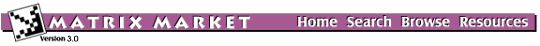## DRIVCAV: Driven cavity

### from the SPARSKIT Collection

Set DRIVCAV
Source: Andrew Chapman, University of Minnesota, chapman@msi.umn.edu
Discipline: Fluid dynamics
Accession: August 1996

These matrices are from modeling 2D fluid flow in a driven cavity. The matrices are non-symmetric and indefinite. They are difficult to solve using iterative methods like preconditioned Krylov subspace methods, because it is difficult to find an effective preconditioner. The intended use of the matrices is for testing iterative solvers.

Preconditioners based on incomplete LU factorization are frequently used for difficult problems, but they are unstable for the matrices in drivcav. This means that the norm of (LU)^{-1} can be extremely large, and the solver will generally fail on the preconditioned system, which may well have a condition number that is worse than that of the unpreconditioned system. Preconditioners like diagonal and SSOR, require inverting diagonal entries. The drivcav matrices have zeros on the diagonal resulting from the incompressibility condition. A way to remove the zeros would be to use a nonsymmetric reordering. Block versions of these preconditioners can also fail due to singular diagonal blocks.

The matrices can be successfully solved using direct methods like frontal or skyline solvers, but as the size of the matrix and the Reynolds number increases, the fillin in the L and U factors increases, and this ultimately limits the use of these solvers.

The physical problem represented by the driven cavity is a square in cross section, with velocity equal to zero on three walls, and equal to one at the fourth wall, in the direction parallel to the fourth wall. This results in a circulating flow, similar to that which would occur in a notch in an infinite flat plate, with the notch cut perpendicular to the free stream flow direction over the plate.

To produce the matrices, the flow was modeled using the incompressible Navier Stokes equations. These were discretized using the Galerkin Finite Element method, and linearized using Newton's method. At Reynolds number equal to zero, the Navier Stokes equations are linear, and Newton's method converges in one iteration. When Reynolds number is increased, depending on the size of the increase, it takes about 4 Newton iterations to converge. The matrices output in the Harwell-Boeing files ExxRyyyy are those for the first Newton iteration after each step increase in Reynolds number.

These matrices supercede the matrices previously referred to in the SPARSKIT collection as DRIVCAV. The original matrices can be found in DRIVCAV_OLD.

Note that the indices in the Harwell-Boeing files are not sorted, that is, in each column in the compressed sparse column format, row entries occur in no particular order.

## Matrices in this set:

The Matrix Market is a service of the Mathematical and Computational Sciences Division / Information Technology Laboratory / National Institute of Standards and Technology.

[ Home ] [ Search ] [ Browse ] [ Resources ]